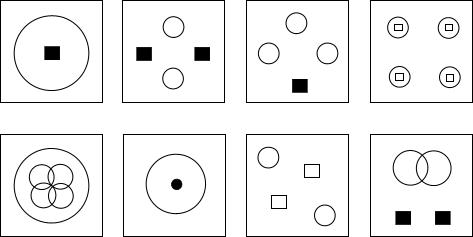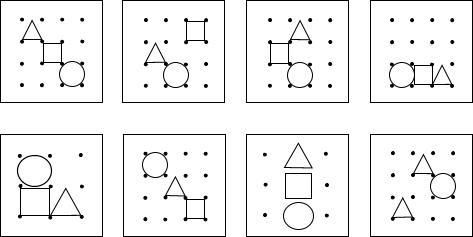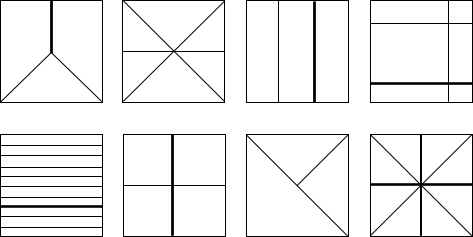EN
Contact Login088 49 59 000

Kantoorgebouw De Blend

Vleutensevaart 100

# How to practise an Assessment?

When you practise your Assessment you want to focus on intelligence tests. The chances of getting the exact same test in your assessment are slim, therefore it is more important to learn how to approach a test question rather than learning a specific test by heart or practising a huge amount of tests. Let’s look at some examples below.## Diagrammatic tests

A common test is the diagrammatic test. Its goal is to recognise similarities or order in a series of diagrammatic figures. Below we will explain several example questions from the LTP and their underlying purposes.One of the figures in the bottom row fits with the upper row and your goal is to select the right one. Do you see the pattern? The correct figure is A, the figure on the left. The answer lies in the realisation that each new figure adds a new circle. So 1 circle, 2 circles, 3 circles, 4 circles and therefore the next figure should include 5 circles. The squares are only there to distract you. Don’t make it more complicated than it has to be. The location of the circles within the figure is apparently not important.

Another good example:Which of the bottom four figures fits with the upper four? You attention will automatically be drawn to the foreground: the circle, the square and the triangle. But they are not the only important thing. Look at the background, do you see it? The grids are 4×4. Therefore two of the four bottom options can immediately be discarded. B and D then remain as the only possible right answers. And then the answer is simple, all upper figures include a circle, a square and a triangle, therefore B is the correct answer. Again it is important not to get distracted by trying to find logic in the movement of the elements within the grid, that is not the key to solve this exercise. As each exercise only has a limited time frame, the time pressure you feel can lead you in the wrong direction.

It is also important to note that the focus in this exercise is not on sequence or order. This is in contrast with the first example we looked at in which a circle was added each time, in this exercise coherence is the key and the order in which the figures appear is irrelevant.

A final example:Which of the figures in the bottom row fits the upper sequence? The first thing you will probably notice is they all contain one bold line. Unfortunately all answers also contain one bold line. So this is not the right approach. You might then decide to count the lines. Most of the figures have three lines (2 normal, 1 bold), only the third figure has two lines. So that is not the key either. Resist the urge to stare at the lines any further. The answer is in the amount of surfaces formed by the lines; either three or six. Which leads to the correct answer straight away: there is only one answer option which contains either three or six surfaces. See how the correct answer does not seem to fit the upper sequence at first glance?

## Number sequences

Number sequences are often part of an intelligence test. They can be real brainteasers. You have to select the next number in a sequence, for example

1 – 4 – 9 – 16 – ?
The correct answer is 25. This is a famous example, each number in the sequence is a square:

1×1, 2×2, 3×3, 4×4.
Therefore the next number has to be the answer to 5×5. This is a rather simple sequence. When you have to add, subtract and multiply in order to recognise the sequence, things will heat up. For example:

3 – 5 – 10 – 8 – 10 – ?
The correct answer is 20. Do you understand why? First you add 2, then you multiply by 2 and then you subtract 2 and then you start again. Therefore you now have to multiply the last number in the sequence (10) by 2. Another possible number sequence might skip a number each time:

1 – 3 – 2 – 6 – 4 – 9 – 8 – 12 – ?
The correct answer here is 16. From 1 to 2 to 4 to 8, the numbers double each time. The numbers in between are only there to distract you, they add 3 each time. They try to trick you with sequences like this. When you practise tests like these, focus on the logic behind the sequences. This will help you recognise how a sequence works and help you achieve high scores on all kinds of number sequence tests.

#### Practice tests are not always similar to the actual tests

Practice tests are often easier than actual tests. At the same time they often lack the element of time pressure. In actual testing the normative group scores are often higher as well. Practice sites try to give you a good feeling about their tests (and therefore about the ads they include). So do not be fooled by practice tests, because scoring well on practice tests does not mean you will get good results on the actual assessment. I know someone who had practised extensively, and held a Cum Laude honours degree, whose test results were mediocre at best. She probably had a case of test fatigue.

#### Cheating the cheater

Make sure you do not end up in a job that is actually above your intelligence level. Because the real challenge is the job itself rather than the assessment test you take. You have to be able to live up to the standard you set with the test.

#### How does practising tests affect the work of assessment agencies?

When you are an assessment psychologist you have to take into account that a candidate will be well prepared when he takes the tests. And that’s ok, as your aim is to establish a candidate’s maximum performance. The combination of test instruments is designed to provide reliable results even when a candidate has practised extensively.